## Example Questions

### Example Question #1 : How To Multiply Monomial Quotients

Find the product: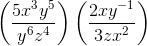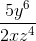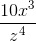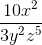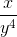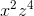Explanation:

Find the product:Step 1: Multiply the numerators and denominators using the properties of exponents. (When multiplying exponents, add them.)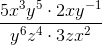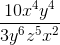Step 2: Simplify the expression.